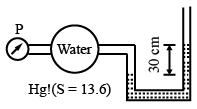# ISRO Scientist or Engineer Mechanical 2010

Instructions

For the following questions answer them individually

Question 71

# If D is the diameter of impeller at inlet, w is the width of impeller at inlet and $$V_f$$ is the velocity of flow at inlet, then discharge through a centrifugal pump is equaltoQuestion 72

# Cavitation parameter is defined byQuestion 73

# The maximum thickness of boundary layerin a pipe of radius R isQuestion 74

# At a point A in a pipe lilne carrying water, the diameter is 1 m, the pressure 98 KPa and the velocity 1 m/s. At a point B, 2 m higher than A, the diameter is 0.5m and the pressure 20 KPa. The direction of flow would beQuestion 75

# In the given figure pressure p in kPa, isQuestion 76

# A small plastic boat loaded with pieces of steel rods is floating in a bathtub. If the cargo is dumped into the water allowing the boat to float empty, the water level in the tub willQuestion 77

# Four cars, with a mass of 1500 kg each, are loaded on a 6 m wide, 12 m long small car ferry. How far, in cm will it sink in the water?Question 78

# Water enters a turbine at 900kPa with negligible velocity. What maximum speed, in m/s, can it reach before it enters the turbine rotor?Question 79

# Given that:Specific gravity of mercury = 13.6;Intensity of pressure = 40kPaExpress the intensity of pressure (gauge) in various units (S.I)Question 80

# The differential equation satisfying $$y = Ae^{3x} + Be^{2x}$$ isOR